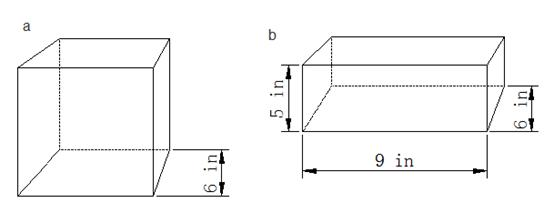### Sample ProblemFigure a is a cube.                                                                   Figure b is a cube.

(a) which figure has a larger surface area?

(b) how much larger? in2

#### Solution

a: 6 in × 6 in × 6 = 216 in2

b: (5 in × 9 in + 9 in × 6 in + 5 in × 6 in) × 2

= 129 in2 × 2

= 258 in2

258 in2 - 216 in2 = 42 in2